SSC CGL Previous Year Questions: Simplification and Square & Cube Root- 2

# SSC CGL Previous Year Questions: Simplification and Square & Cube Root- 2 - SSC CGL

Test Description

## 30 Questions MCQ Test SSC CGL (Tier - 1) - Previous Year Papers (Topic Wise) - SSC CGL Previous Year Questions: Simplification and Square & Cube Root- 2

SSC CGL Previous Year Questions: Simplification and Square & Cube Root- 2 for SSC CGL 2023 is part of SSC CGL (Tier - 1) - Previous Year Papers (Topic Wise) preparation. The SSC CGL Previous Year Questions: Simplification and Square & Cube Root- 2 questions and answers have been prepared according to the SSC CGL exam syllabus.The SSC CGL Previous Year Questions: Simplification and Square & Cube Root- 2 MCQs are made for SSC CGL 2023 Exam. Find important definitions, questions, notes, meanings, examples, exercises, MCQs and online tests for SSC CGL Previous Year Questions: Simplification and Square & Cube Root- 2 below.
Solutions of SSC CGL Previous Year Questions: Simplification and Square & Cube Root- 2 questions in English are available as part of our SSC CGL (Tier - 1) - Previous Year Papers (Topic Wise) for SSC CGL & SSC CGL Previous Year Questions: Simplification and Square & Cube Root- 2 solutions in Hindi for SSC CGL (Tier - 1) - Previous Year Papers (Topic Wise) course. Download more important topics, notes, lectures and mock test series for SSC CGL Exam by signing up for free. Attempt SSC CGL Previous Year Questions: Simplification and Square & Cube Root- 2 | 30 questions in 30 minutes | Mock test for SSC CGL preparation | Free important questions MCQ to study SSC CGL (Tier - 1) - Previous Year Papers (Topic Wise) for SSC CGL Exam | Download free PDF with solutions
 1 Crore+ students have signed up on EduRev. Have you?
SSC CGL Previous Year Questions: Simplification and Square & Cube Root- 2 - Question 1

### The sum of four numbers is 48. When 5 and 1 are added to the first two; and 3 & 7 are subtracted from the 3rd & 4th, the numbers will be equal. The numbers are        (SSC CGL 1st Sit. 2015)

Detailed Solution for SSC CGL Previous Year Questions: Simplification and Square & Cube Root- 2 - Question 1

Let four numbers are a, b, c, d, then
a + b + c + d = 48 ...(i)
and a + 5 = b + 1 ...(ii)
or, a = b – 4 ...(iii)
and c – 3 = d – 7 ...(iv)
c = d – 4 ...(v)
Substituting equation (iii) and (v) in equation (i) we get
b – 4 + b + d – 4 + d = 48
b + d = 28 ...(vi)
But we know,
b + 1 = d – 7
∴ b = d – 8
Substituting in equation (vi) we get
d – 8 + d = 28
d = 18
Solving this way we get a = 6, b = 10, c = 14 and d = 18

SSC CGL Previous Year Questions: Simplification and Square & Cube Root- 2 - Question 2

### In an exam the sum  of the scores of A and B is 120, that of B and C is 130 and that of C and A is 140. Then the score of C is:       (SSC CHSL 2015)

Detailed Solution for SSC CGL Previous Year Questions: Simplification and Square & Cube Root- 2 - Question 2

A + B = 120 … (i)
B + C = 130 … (ii)
C + A = 140 …(iii)
2A + 2B + 2C = 390
A + B + C = 195
But, A + B = 120
So, C = 195 – 120 = 75

SSC CGL Previous Year Questions: Simplification and Square & Cube Root- 2 - Question 3

### The simplified value of following is: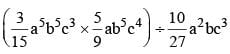(SSC CHSL 2015)

Detailed Solution for SSC CGL Previous Year Questions: Simplification and Square & Cube Root- 2 - Question 3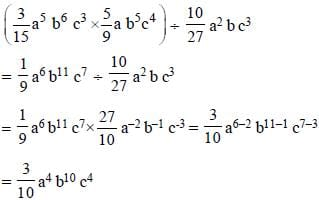SSC CGL Previous Year Questions: Simplification and Square & Cube Root- 2 - Question 4

If 32x -y = 3x +y = √27, then the value of  3x -y will be :        (SSC Sub. Ins. 2015)

Detailed Solution for SSC CGL Previous Year Questions: Simplification and Square & Cube Root- 2 - Question 4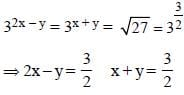4x – 2y = 3 ...(i)
2x + 2y = 3 ...(ii)
Solving equation (i) and (ii)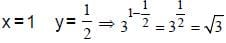SSC CGL Previous Year Questions: Simplification and Square & Cube Root- 2 - Question 5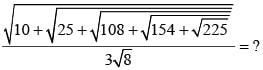(SSC Sub. Ins. 2015)

Detailed Solution for SSC CGL Previous Year Questions: Simplification and Square & Cube Root- 2 - Question 5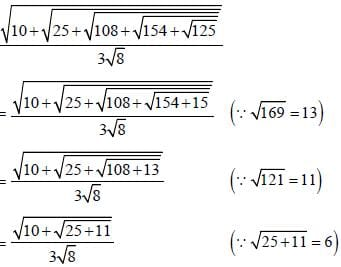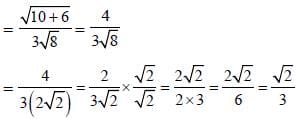SSC CGL Previous Year Questions: Simplification and Square & Cube Root- 2 - Question 6

The simplified value of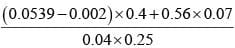is:       (SSC Sub. Ins. 2015)

Detailed Solution for SSC CGL Previous Year Questions: Simplification and Square & Cube Root- 2 - Question 6

0.0539 – 0.002 = 0.0519
0.56 × 0.07 = 0.0392
0.0519 × 0.4 = 0.02076
0.04 × 0.25 = 0.01
So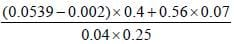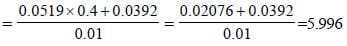SSC CGL Previous Year Questions: Simplification and Square & Cube Root- 2 - Question 7

2km 5m is equal to:       (SSC Sub. Ins. 2015)

Detailed Solution for SSC CGL Previous Year Questions: Simplification and Square & Cube Root- 2 - Question 7

2km 5m = 2 + (5/100) = 2.005 km

SSC CGL Previous Year Questions: Simplification and Square & Cube Root- 2 - Question 8

Arrange the following in ascending order 334, 251, 717, we get        (SSC CGL 1st Sit. 2014)

Detailed Solution for SSC CGL Previous Year Questions: Simplification and Square & Cube Root- 2 - Question 8

334 = (32)17 = 917
251 = (23)17 = 817
717 > 817 > 917
or 717 > 251 > 334

SSC CGL Previous Year Questions: Simplification and Square & Cube Root- 2 - Question 9

Which one of the following is true?       (SSC CHSL 2014)

Detailed Solution for SSC CGL Previous Year Questions: Simplification and Square & Cube Root- 2 - Question 9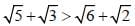Squaring both sides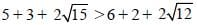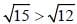which is true.

SSC CGL Previous Year Questions: Simplification and Square & Cube Root- 2 - Question 10

Ram left 1/3 of his property  to his widow and 3/5 of theremainder to his daughter. He gave the rest to his son who received ₹ 6,400. How much was his original property worth?       (SSC CHSL 2014)

Detailed Solution for SSC CGL Previous Year Questions: Simplification and Square & Cube Root- 2 - Question 10

Let original property worth ₹ x
Property left for Ram’s widow = x/3
Property left for his daughter =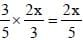Remaining property =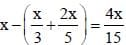SSC CGL Previous Year Questions: Simplification and Square & Cube Root- 2 - Question 11

The simplified value of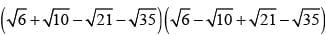is        (SSC Sub. Ins. 2014)

Detailed Solution for SSC CGL Previous Year Questions: Simplification and Square & Cube Root- 2 - Question 11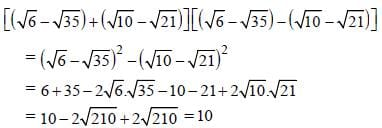SSC CGL Previous Year Questions: Simplification and Square & Cube Root- 2 - Question 12

The next term of the sequence,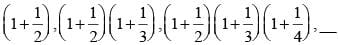is         (SSC Sub. Ins. 2014)

Detailed Solution for SSC CGL Previous Year Questions: Simplification and Square & Cube Root- 2 - Question 12

Next term will be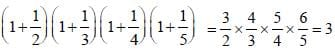SSC CGL Previous Year Questions: Simplification and Square & Cube Root- 2 - Question 13

If '+' means ' ÷ ', '×' means '–', ' ÷ ' means '×' and '–' means '+', what will be the value of the following expression?
9 + 3 ÷ 4 – 8 × 2 = ?          (SSC Sub. Ins. 2014)

Detailed Solution for SSC CGL Previous Year Questions: Simplification and Square & Cube Root- 2 - Question 13

9 + 3 ÷ 4 – 8 × 2 = ?
Applying rules
9 ÷ 3 × 4 + 8 – 2 = ?
3 × 4 + 8 – 2 = ?
20 – 2 = ?
? = 18

SSC CGL Previous Year Questions: Simplification and Square & Cube Root- 2 - Question 14

The value of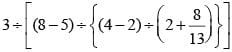is          (SSC Sub. Ins. 2014)

Detailed Solution for SSC CGL Previous Year Questions: Simplification and Square & Cube Root- 2 - Question 14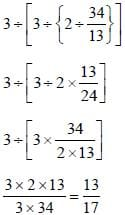SSC CGL Previous Year Questions: Simplification and Square & Cube Root- 2 - Question 15

Evaluate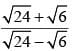(SSC Sub. Ins. 2014)

Detailed Solution for SSC CGL Previous Year Questions: Simplification and Square & Cube Root- 2 - Question 15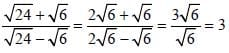SSC CGL Previous Year Questions: Simplification and Square & Cube Root- 2 - Question 16

If (23)2 = 4x then 3x is equal to        (SSC CGL 2nd Sit. 2013)

Detailed Solution for SSC CGL Previous Year Questions: Simplification and Square & Cube Root- 2 - Question 16

(23)2 = 4x
26 = 22x
6 = 2x
x = 3
33 = 27

SSC CGL Previous Year Questions: Simplification and Square & Cube Root- 2 - Question 17

Which one of the following is the minimum value of the sum of two integers whose product is 24?       (SSC CGL 2nd Sit. 2013)

Detailed Solution for SSC CGL Previous Year Questions: Simplification and Square & Cube Root- 2 - Question 17

Product of 2 no is = 24
Possible pair of factor = (1, 24) (2, 12) (3, 8) (4, 6) i,e., 4 + 6 is minimum = 10

SSC CGL Previous Year Questions: Simplification and Square & Cube Root- 2 - Question 18

The value of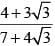is        (SSC CGL 2nd Sit. 2013)

Detailed Solution for SSC CGL Previous Year Questions: Simplification and Square & Cube Root- 2 - Question 18

Expression =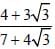Rationalising the denominator.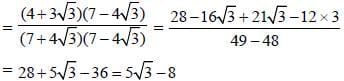SSC CGL Previous Year Questions: Simplification and Square & Cube Root- 2 - Question 19

The greatest 4 digit member which is a perfect square, is         (SSC CGL 2nd Sit. 2013)

Detailed Solution for SSC CGL Previous Year Questions: Simplification and Square & Cube Root- 2 - Question 19

99 × 99 = 9801
"Alternate Method:
1002 = 10000 which is a 5 digits number
Hence, 992 is required number."

SSC CGL Previous Year Questions: Simplification and Square & Cube Root- 2 - Question 20

The fourth root of 24010000 is       (SSC CGL 2nd Sit. 2013)

Detailed Solution for SSC CGL Previous Year Questions: Simplification and Square & Cube Root- 2 - Question 20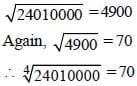SSC CGL Previous Year Questions: Simplification and Square & Cube Root- 2 - Question 21

The smallest positive integer which when multiplied by 392, gives a perfect square is       (SSC CGL 2nd Sit. 2013)

Detailed Solution for SSC CGL Previous Year Questions: Simplification and Square & Cube Root- 2 - Question 21

392 × 2 = 784 ⇒ (28)2
Hence, 2 can be multiplied by 392 which gives perfect square.

SSC CGL Previous Year Questions: Simplification and Square & Cube Root- 2 - Question 22

If a = 2, b = 3, then (ab + ba)–1 is       (SSC CGL 2nd Sit. 2013)

Detailed Solution for SSC CGL Previous Year Questions: Simplification and Square & Cube Root- 2 - Question 22

(ab + ba)–1 = (23 + 32)–1 = (8 + 9)–1 = (17)–1 = 1/17

SSC CGL Previous Year Questions: Simplification and Square & Cube Root- 2 - Question 23

If x2 = y + z, y2 = z + x and z2 = x + y, then the value of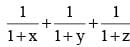is        (SSC CGL 2013)

Detailed Solution for SSC CGL Previous Year Questions: Simplification and Square & Cube Root- 2 - Question 23

x2 = y + z
⇒ x2 + x = x + y + z
⇒ x (x + 1) = x + y + z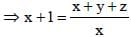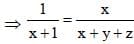Similarly,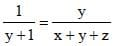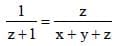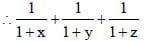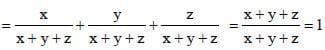SSC CGL Previous Year Questions: Simplification and Square & Cube Root- 2 - Question 24

The numerator of a fraction is 4 less than its denominator. If the numerator is decreased by 2 and the denominator is increased by 1, then the denominator becomes eight times the numerator. Find the fraction.         (SSC CGL 2013)

Detailed Solution for SSC CGL Previous Year Questions: Simplification and Square & Cube Root- 2 - Question 24

Original fraction =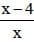In case II,
8 (x – 4 – 2) = x + 1
⇒ 8x – 48 = x + 1
⇒ 7x = 49 ⇒ x = 7
Original fraction =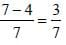SSC CGL Previous Year Questions: Simplification and Square & Cube Root- 2 - Question 25

Find the simplest value of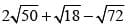(given √2 = 1.414 ).        (SSC CGL 2013)

Detailed Solution for SSC CGL Previous Year Questions: Simplification and Square & Cube Root- 2 - Question 25

Expression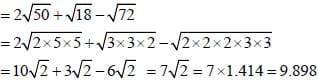SSC CGL Previous Year Questions: Simplification and Square & Cube Root- 2 - Question 26

A rational number between 3/4 and 3/8 is        (SSC CGL 2013)

Detailed Solution for SSC CGL Previous Year Questions: Simplification and Square & Cube Root- 2 - Question 26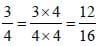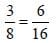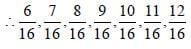∴ Required rational number = 9/16.

SSC CGL Previous Year Questions: Simplification and Square & Cube Root- 2 - Question 27

Number of digits in the square root of 62478076 is :       (SSC CGL 2013)

Detailed Solution for SSC CGL Previous Year Questions: Simplification and Square & Cube Root- 2 - Question 27

When no. of digit in a no. is 7 or 8 then in square root will be 4.

SSC CGL Previous Year Questions: Simplification and Square & Cube Root- 2 - Question 28

If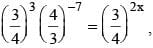then x is :        (SSC CGL 1st Sit. 2013)

Detailed Solution for SSC CGL Previous Year Questions: Simplification and Square & Cube Root- 2 - Question 28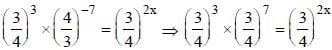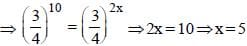SSC CGL Previous Year Questions: Simplification and Square & Cube Root- 2 - Question 29

If a number is as much greater than 31 as it is less than 75, then the number is.         (SSC CHSL 2013)

Detailed Solution for SSC CGL Previous Year Questions: Simplification and Square & Cube Root- 2 - Question 29

x – 31 = 75 – x
2x = 106
x = 53

SSC CGL Previous Year Questions: Simplification and Square & Cube Root- 2 - Question 30

Find the value of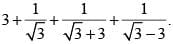(SSC CHSL 2013)

Detailed Solution for SSC CGL Previous Year Questions: Simplification and Square & Cube Root- 2 - Question 30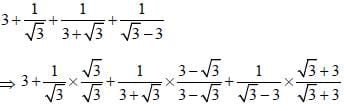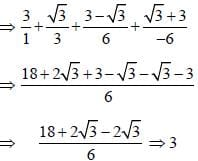## SSC CGL (Tier - 1) - Previous Year Papers (Topic Wise)

250 tests
Information about SSC CGL Previous Year Questions: Simplification and Square & Cube Root- 2 Page
In this test you can find the Exam questions for SSC CGL Previous Year Questions: Simplification and Square & Cube Root- 2 solved & explained in the simplest way possible. Besides giving Questions and answers for SSC CGL Previous Year Questions: Simplification and Square & Cube Root- 2, EduRev gives you an ample number of Online tests for practice

250 tests